Number Line, -1 to 1 in Tenths

Number line for classroom activities.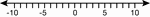Number Line, -10 to 10 by Fives

Number line for classroom activities.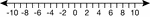Number Line, -10 to 10 by Twos

Number line for classroom activities.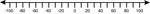Number Line, -100 to 100 by 20s

Number line for classroom activities.Number Line, -100 to 100 by Fives

Number line for classroom activities.Number Line, -1000 to 1000 by 100s

Number line for classroom activities.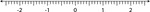Number Line, -2 to 2 in Tenths

Number line for classroom activities.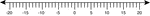Number Line, -20 to 20

Number line for classroom activities.Number Line, -25 to 25

Number line for classroom activities.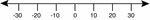Number Line, -30 to 30 by Tens

Number line for classroom activities.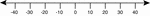Number Line, -40 to 40 by Tens

Number line for classroom activities.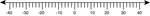Number Line, -40 to 40 by Twos

Number line for classroom activities.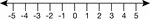Number Line, -5 to 5

Number line for classroom activities.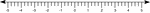Number Line, -5 to 5 in Fourths

Number line for classroom activities.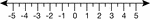Number Line, -5 to 5 in Halves

Number line for classroom activities.Number Line, -5 to 5 in Tenths

Number line for classroom activities.Number Line, -5 to 5 in Tenths

Number line for classroom activities.Number Line, -50 to 50

Number line for classroom activities.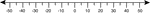Number Line, -50 to 50 by Fives

Number line for classroom activities.Number Line, -50 to 50 by Fives

Number line for classroom activities.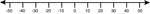Number Line, -50 to 50 by Tens

Number line for classroom activities.Number Line, -75 to 75 by Fives

Number line for classroom activities.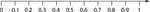Number Line, 0 to 1 by Tenths

Number line for classroom activities.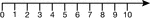Number Line, 0-10

Number line for classroom activities.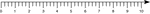Number Line, 0-10 in Fourths

Number line for classroom activities.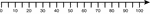Number Line, 0-100 by Tens

Number line for classroom activities.Number Line, 0-25

Number line for classroom activities.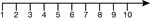Number Line, 1-10

Number line for classroom activities.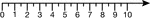Number Line, 1-10 in Halves

Number line for classroom activities.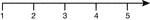Number Line, 1-5

Number line for classroom activities.